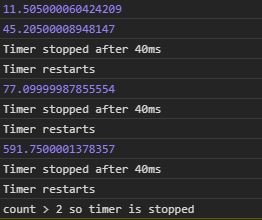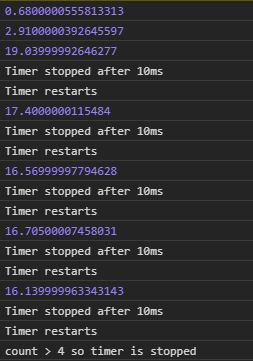Open in App
Not now

# D3.js timer.restart() Function

• Last Updated : 29 Jul, 2020

The timer.restart() function in D3.js is used to restart a timer with the given function and delay. The timer.restart() function is used when one wants to reset the timer and start again.

Syntax:

```timer.restart(callback, delay);
```

Parameters: It takes two parameters as mentioned above and described below:

• callback: It is the function to be stopped or start after a particular delay.
• delay: It is the time after which the function will be executed or stop

Example 1: When no delay is given.

## HTML

 ```<``html` `lang``=``"en"``>`` ` `<``head``>``    ``<``meta` `charset``=``"UTF-8"``>``    ``<``meta` `name``=``"viewport"` `content``=``        ``"width=device-width, initial-scale=1.0"``>```` ` `<``body``>``    ````    ``<``script` `type``=``"text/javascript"` `        ``src``=``"https://d3js.org/d3.v4.min.js"``>``    ````     ` `    ``<``script``>``        ``count = 0;``        ``let func = function (e) {``            ``console.log(e)``            ``if (e > 40) {``                ``console.log("Timer stopped after 40ms")``                ``if (e > 40) {``                    ``count++;`` ` `                    ``// Restarting the timer again``                    ``console.log("Timer restarts")``                    ``timer.restart(func)``                ``}``                ``if (count > 2) {``                    ``timer.stop();``                    ``console.log(``                        ``"count > 2 so timer is stopped")``                ``}``            ``}``        ``}``        ``var timer = d3.timer(func);``    `````` ` ``

Output:Example 2: When a delay is given.

## HTML

 ```<``html` `lang``=``"en"``>`` ` `<``head``>``    ``<``meta` `charset``=``"UTF-8"``>``    ``<``meta` `name``=``"viewport"` `content``=``        ``"width=device-width, initial-scale=1.0"``>```` ` `<``body``>``    ````    ``<``script` `type``=``"text/javascript"` `        ``src``=``"https://d3js.org/d3.v4.min.js"``>``    ````     ` `    ``<``script``>``        ``count = 0;``        ``let func = function (e) {``            ``console.log(e)``            ``if (e > 10) {``                ``console.log("Timer stopped after 10ms")``                ``if (e > 10) {``                    ``count++;`` ` `                    ``// Restarting the timer again``                    ``console.log("Timer restarts")``                    ``timer.restart(func)``                ``}``                ``if (count > 4) {``                    ``timer.stop();``                    ``console.log(``                    ``"count > 4 so timer is stopped")``                ``}``            ``}``        ``}``        ``// A delay of 2000ms``        ``var timer = d3.timer(func, 2000);``    `````` ` ``

Output:My Personal Notes arrow_drop_up